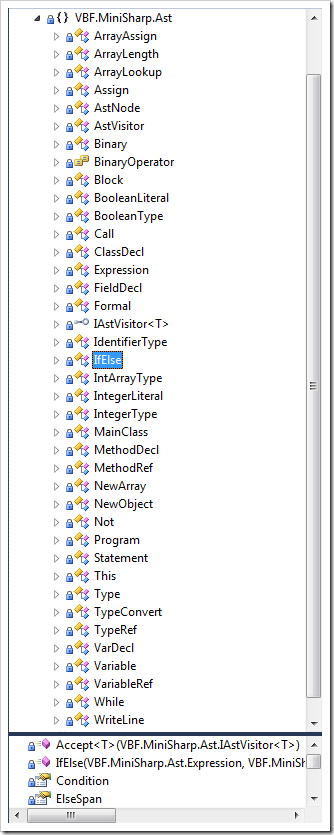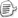miniSharp语言是C#的一个小子集，然而它仍然具有一门完整编程语言的所有要素，而且仍然是一种面向对象的语言。我们把miniSharp的语法分成三类——声明结构、语句和表达式。声明结构就是类、方法、字段的声明。语句就是诸如if-else、while这样特定含义的指令。而表达式则是表示一种有运算结果的结构，如二元运算符表达式、函数调用表达式等。C#中赋值也是一种表达式，但miniSharp为了简化后续代码生成，将赋值当成一种语句。

 Program → MainClass ClassDecl* MainClass → static class ID { public static void Main (string[] ID){ Statement+ } } ClassDecl → class ID { FieldDecl* MethodDecl* } → class ID : ID { FieldDecl* MethodDecl* } FieldDecl → Type ID; MethodDecl → public Type ID (FormalList) { Statement* return Exp ; } FormalList → Type ID FormalRest* → ε FormalRest → , Type ID Type → int[] → bool → int → ID

 Statement → { Statement* } → if ( Exp ) Statement else Statement → while ( Exp ) Statement → System.Console.WriteLine( Exp ) → ID = Exp ; → ID [ Exp ] = Exp ; → Type ID ;

 Exp → Exp op Exp → Exp[ Exp ] → Exp .Length → Exp .ID ( ExpList ) → INTEGER_LITERAL → true → false → ID → this → new int [ Exp ] → new ID () → ! Exp → ( Exp ) ExpList → Exp ExpRest* → ε ExpRest → , Exp

 1 (Exp)  new this 变量 常量方法调用 属性访问 数组访问 2 ! 3 * / 4 + - 5 < > == 6 && 7 ||

 BasicExp → 括号、new、this、变量、常量、方法调用、属性访问、数组访问 Factor → BasicExp → ! Factor Term → Factor → Term op Factor   其中 op 是 * / Comparand → Term → Comparand op Term   其中 op 是 + - Comparison → Comparand → Comparison op Comparand    其中 op 是 < > == Logical → Comparison → Logical && Comparison Exp → Logical → Exp || Logicalpublic class IfElse : Statement { public Expression Condition { get; private set; } public Statement TruePart { get; private set; } public Statement FalsePart { get; private set; } public SourceSpan IfSpan { get; private set; } public SourceSpan ElseSpan { get; private set; } public IfElse(Expression cond, Statement truePart, Statement falsePart, SourceSpan ifSpan, SourceSpan elseSpan) { Condition = cond; TruePart = truePart; FalsePart = falsePart; IfSpan = ifSpan; ElseSpan = elseSpan; } public override T Accept(IAstVisitor visitor) { return visitor.VisitIfElse(this); } } 

 PProgram.Reference = // MainClass ClassDecl* from main in PMainClass from classes in PClassDecl.Many() select new Program(main, classes); PMainClass.Reference = // static class id { public static void Main(string[] id) { statement }} from _static1 in K_STATIC from _class in K_CLASS from className in ID from _1 in LEFT_BR from _public in K_PUBLIC from _static2 in K_STATIC from _void in K_VOID from _main in K_MAIN from _2 in LEFT_PH from _string in K_STRING from _3 in LEFT_BK from _4 in RIGHT_BK from arg in ID from _5 in RIGHT_PH from _6 in LEFT_BR from statements in PStatement.Many1() from _7 in RIGHT_BR from _8 in RIGHT_BR select new MainClass(className, arg, statements); 

 var paramFormal = from paramType in PType from paramName in ID select new Formal(paramType, paramName); PFormalList.Reference = // Type id FormalRest* | (from first in paramFormal from rest in PFormalRest.Many() select new[] { first }.Concat(rest).ToArray()) | Parsers.Succeed(new Formal); PFormalRest.Reference = // , Type id paramFormal.PrefixedBy(COMMA.AsParser()); 

 var termRest = from op in (ASTERISK.AsParser() | SLASH.AsParser()) from factor in PFactor select new { Op = op, Right = factor }; PTerm.Reference = // term * factor | factor from factor in PFactor from rest in termRest.Many() select rest.Aggregate(factor, (f, r) => new Binary(r.Op, f, r.Right)); var comparandRest = from op in (PLUS.AsParser() | MINUS.AsParser()) from term in PTerm select new { Op = op, Right = term }; PComparand.Reference = // comparand + term | term from term in PTerm from rest in comparandRest.Many() select rest.Aggregate(term, (t, r) => new Binary(r.Op, t, r.Right)); var comparisonRest = from op in (LESS.AsParser() | GREATER.AsParser() | EQUAL.AsParser()) from comparand in PComparand select new { Op = op, Right = comparand }; PComparison.Reference = // comparison < comparand | comparand from comparand in PComparand from rest in comparisonRest.Many() select rest.Aggregate(comparand, (c, r) => new Binary(r.Op, c, r.Right));posted on 2011-07-08 01:19  装配脑袋  阅读(14843)  评论(23编辑  收藏  举报